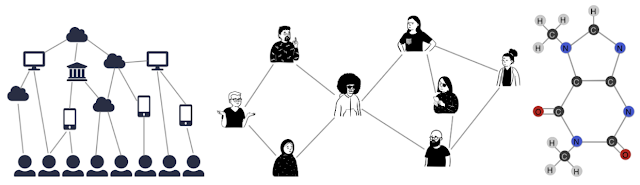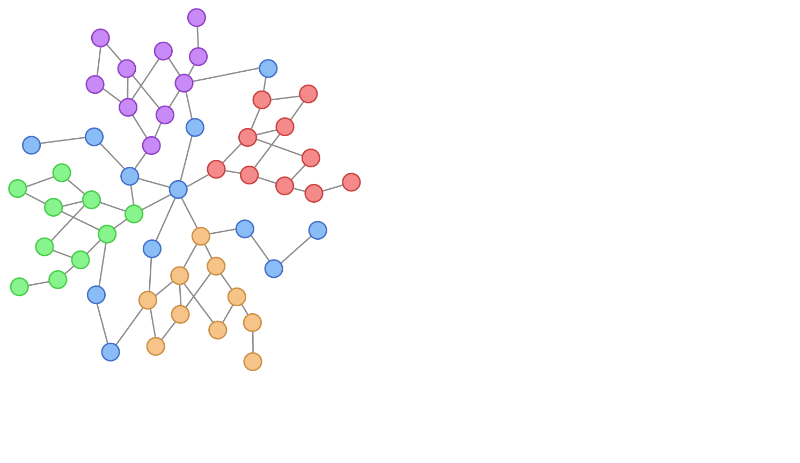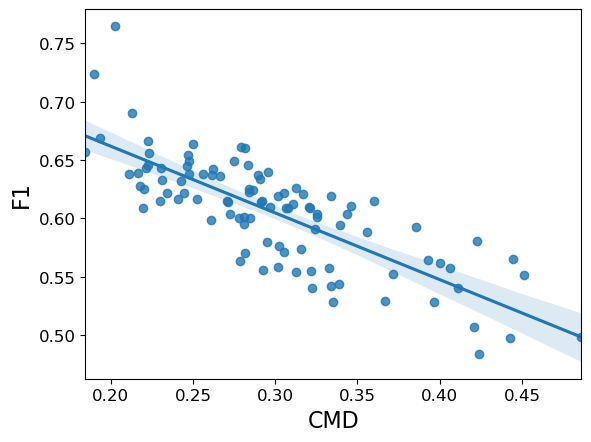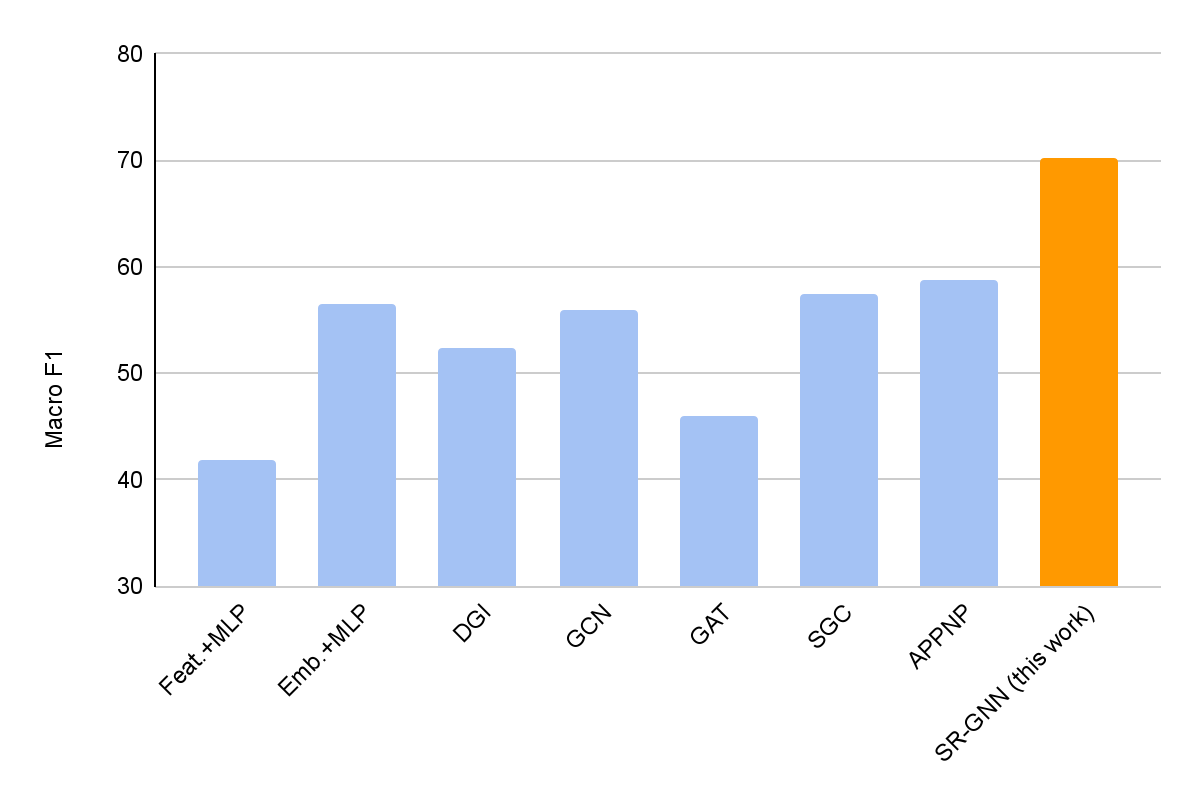# Robust Graph Neural Networks

Graph Neural Networks (GNNs) are powerful tools for leveraging graph-structured data in machine learning. Graphs are flexible data structures that can model many different kinds of relationships and have been used in diverse applications like traffic prediction, rumor and fake news detection, modeling disease spread, and understanding why molecules smell.Graphs can model the relationships between many different types of data, including web pages (left), social connections (center), or molecules (right).

As is standard in machine learning (ML), GNNs assume that training samples are selected uniformly at random (i.e., are an independent and identically distributed or “IID” sample). This is easy to do with standard academic datasets, which are specifically created for research analysis and therefore have every node already labeled. However, in many real world scenarios, data comes without labels, and labeling data can be an onerous process involving skilled human raters, which makes it difficult to label all nodes. In addition, biased training data is a common issue because the act of selecting nodes for labeling is usually not IID. For example, sometimes fixed heuristics are used to select a subset of data (which shares some characteristics) for labeling, and other times, human analysts individually choose data items for labeling using complex domain knowledge.Localized training data is a typical non-IID bias exhibited in graph-structured data. This is shown on the left figure by taking an orange node and expanding to those around it. Instead, an IID training sample of nodes for labeling would be uniformly distributed, as illustrated by the sampling process on the right.

To quantify the amount of bias present in a training set, one can use methods that measure how large the shift is between two different probability distributions, where the size of the shift can be thought of as the amount of bias. As the shift grows in size, machine learning models have more difficulty generalizing from the biased training set. This situation can meaningfully hurt generalizability — on academic datasets, we’ve observed domain shifts causing a performance drop of 15-20% (as measured by the F1 score).

In “Shift-Robust GNNs: Overcoming the Limitations of Localized Graph Training Data”, presented at NeurIPS 2021, we introduce a solution for using GNNs on biased data. Called Shift-Robust GNN (SR-GNN), this approach is designed to account for distributional differences between biased training data and a graph’s true inference distribution. SR-GNN adapts GNN models to the presence of distributional shift between the nodes labeled for training and the rest of the dataset. We illustrate the effectiveness of SR-GNN in a variety of experiments with biased training datasets on common GNN benchmark datasets for semi-supervised learning and show that SR-GNN outperforms other GNN baselines in accuracy, reducing the negative effects of biased training data by 30–40%.

The Impact of Distribution Shifts on Performance
To demonstrate how distribution shift affects GNN performance, we first generate a number of biased training sets for known academic datasets. Then in order to understand the effect, we plot the generalization (test accuracy) versus a measure of distribution shift (the Central Moment Discrepancy1, CMD). For example, consider the well known PubMed citation dataset, which can be thought of as a graph where the nodes are medical research papers and the edges represent citations between them. When we generate biased training data for PubMed, the plot looks like this:The effect of distribution shift on the PubMed dataset. Performance (F1) is shown on the y-axis vs. the distribution shift, Central Moment Discrepancy (CMD), on the x-axis, for 100 biased training set samples. As the distribution shift increases, the model’s accuracy falls.

Here one can observe a strong negative correlation between the distribution shift in the dataset and the classification accuracy: as CMD increases, the performance (F1) decreases. That is, GNNs can have difficulty generalizing as their training data looks less like the test dataset.

To address this, we propose a shift-robust regularizer (similar in idea to domain-invariant learning) to minimize the distribution shift between training data and an IID sample from unlabeled data. To do this, we measure the domain shift (e.g., via CMD) in real time as the model is training and apply a direct penalty based on this that forces the model to ignore as much of the training bias as possible. This forces the feature encoders that the model learns for the training data to also work effectively for any unlabeled data, which might come from a different distribution.

The figure below shows what this looks like when compared to a traditional GNN model. We still have the same inputs (the node features X, and the Adjacency Matrix A), and the same number of layers. However at the final embedding Zk from layer (k) of the GNN is compared against embeddings from unlabeled data points to verify that the model is correctly encoding them.SR-GNN adds two kinds of regularizations to deep GNN models. First, a domain shift regularization (λ term) minimizes the distance between hidden representations of the labeled (Zk) and unlabeled (ZIID) data. Second, the instance weight (β) of the examples can be changed to further approximate the true distribution.

We write this regularization as an additional term in the formula for the model’s loss based on the distance between the training data’s representations and the true data’s distribution (full formulas available in the paper).

In our experiments, we compare our method and a number of standard graph neural network models, to measure their performance on node classification tasks. We demonstrate that adding the SR-GNN regularization gives a 30–40% percent improvement on classification tasks with biased training data labels.A comparison of SR-GNN using node classification with biased training data on the PubMed dataset. SR-GNN outperforms seven baselines, including DGI, GCN, GAT, SGC and APPNP.

## Shift-Robust Regularization for Linear GNNs via Instance Re-weighting

Moreover, it’s worth noting that there’s another class of GNN models (e.g., APPNP, SimpleGCN, etc) that are based on linear operations to speed up their graph convolutions. We also examined how to make these models more reliable in the presence of biased training data. While the same regularization mechanism can not be directly applied due to their different architecture, we can “correct” the training bias by re-weighting the training instances according to their distance from an approximated true distribution. This allows correcting the distribution of the biased training data without passing gradients through the model.

Finally, the two regularizations — for both deep and linear GNNs — can be combined into a generalized regularization for the loss, which combines both domain regularization and instance reweighting (details, including the loss formulas, available in the paper).

## Conclusion

Biased training data is common in real world scenarios and can arise due to a variety of reasons, including difficulties of labeling a large amount of data, the various heuristics or inconsistent techniques that are used to choose nodes for labeling, delayed label assignment, and others. We presented a general framework (SR-GNN) that can reduce the influence of biased training data and can be applied to various types of GNNs, including both deeper GNNs and more recent linearized (shallow) versions of these models.

## Acknowledgements

Qi Zhu is a PhD Student at UIUC. Thanks to our collaborators Natalia Ponomareva (Google Research) and Jiawei Han (UIUC). Thanks to Tom Small and Anton Tsitsulin for visualizations.

1We note that many measures of distribution shift have been proposed in the literature. Here we use CMD (as it is quick to calculate and generally shows good performance in the domain adaptation literature), but the concept generalizes to any measure of distribution distances/domain shift.